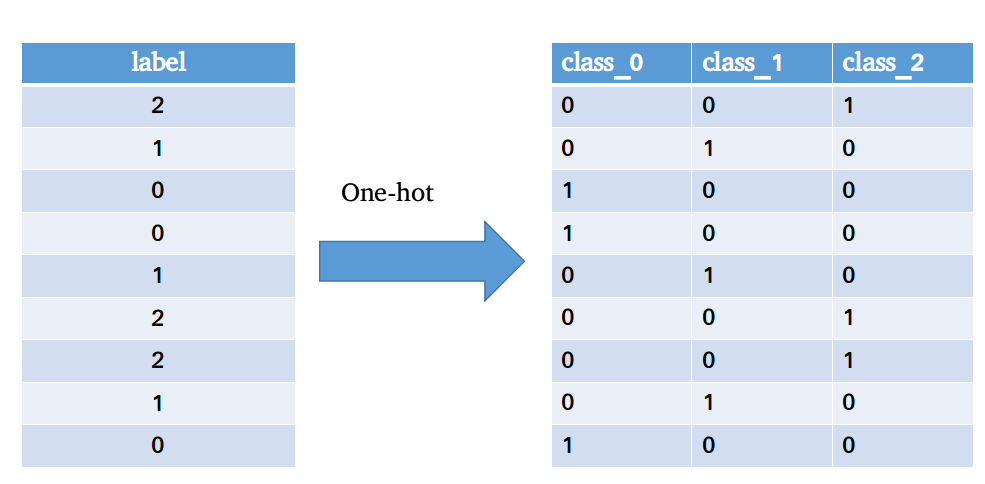# python-一些骚的要命的操作。

## 1.交换字典的键与值。

{‘name':'sam','sex':'male'}  ----> {'sam':name','male':sex'}

dict(zip(dictionary.values(), dictionary.keys()))

## 2.one-hot编码（机器学习算法相关）Y = np.zeros((n_sample, n_class), dtype=np.float64)
for k in range(n_class):
Y[:, k] = y == k